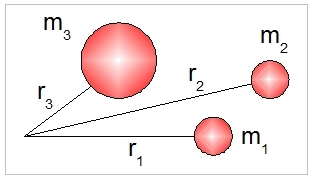# Rotation

## Moment of inertia

Consider a rigid body that rotates about a given axis with an angular velocity of ω. It can be shown that:

τ  = I . α

where:
τ  : Torque (couple) (Nm)
I : Moment of inertia (kg . m2)
α : Angular acceleration (rad / s2)

The moment of inertia I depends on the shape of the object and its distribution of pointmasses that build up the object. The moment of inertia can be calculated from:
I = Summation [point masses . (r2)].

where:
r : Radius measured from the axis of rotation.## Energy of rotating object

The energy stored in a rotating body equals:

E = 1/2 . I . ω2

where:
E : Energy (J)
I : Moment of Inertia (kg . m2)
ω : Angular velocity (rad / s)

## Torque and power

The power needed to rotate an object by applying torque τ with a resulting angular speed of ω is:
P = τ  . ω

with:
P : Power (Watt)
τ  : Torque (N.m)
ω : Angular speed (rad / s)

## Explanation

### Moment of inertia

We explain the relevance of the formula τ = I . α by looking at the analogy of the formula F = m . a, which we know from linear motion theory (Newton's Second Law). The moment of inertia is, thus, explained as the ability to resist a change in rotational motion. This means that a rotating object will stay rotating at the same rotational speed as long as there are no working moments on the object.

The formula for the moment of inertia I highlights that it depends on the distribution of point masses in a body, relative to the axis of rotation. The greater the distance of point masses from the axis of rotation, the greater its distribution to the moment of inertia. The moment of inertia, clearly, depends on shape and the position of the axis of rotation.

The following table gives the moment of inertia for some common bodies:

 Object Moment of inertia Massive cylinder, cylinder axis 1/2 . m . R2 Thin rod, axis midway 1/12 . m . l2 Thin disk, traverse axis through centre 1/4 . m . R2

### Energy of rotating object

The formula for rotational energy also looks familiar, because it is analogous to the formula for the kinetic energy of a linear moving object. The stored rotational energy of an object depends on both the moment of inertia and the angular velocity.

### Torque and power

The energy needed to rotate an object follows on from the definition of work: W = F . d. For a rotating object, we get E = τ  . θ. Note that torque already has the unit N.m.
The power is given by P = E / T = τ  . θ / T = τ  . ω.

## Examples

### Moment of inertia

A rod with a length of 10 metres is rotating about an axis positioned midway. The mass of the rod is 10 kg. What is the moment of inertia of the rod in this situation?

I = (1/12) . m . L2  = (1/12) . 10 . 100 =1000 / 12 = 83,3 kg.m2

We now start rotating this rod by applying a torque of 400 Nm. How long will it take for this rod to reach a speed of 500 rpm (revolutions per minute)?
τ  = I . α
400 = 83,3α
α =  4.8 rad / s2

ω = α . t
2π.500 / 60 = 4.8 t
100π/6 = 4.8t
t = 10,9 seconds

### Energy of rotating object

How much energy is stored in the rotating rod in the example above?

E = 1/2 . I . ω2 = 1/2 . 83,3 . (100π/6 )2 =  114.1 KJ

### Torque and power

A rotor system is turning at a speed of 500 rpm. To sustain this rotational speed a torque of 400 Nm is required. What power is needed to supply the rotor system?

P = τ  . ω = 400 . 500 . 2π/ 60 = 4,000π/6 =  20.9 KW.

# Do you want to comment this topic?

1: (Book) Cyclic and Collective2: (Book) Principles of Helicopter Flight3: Microsoft FSX Steam Edition4: Logitech Extreme 3D Pro Joystick5: Saitek Pro Flight Rudder Pedals